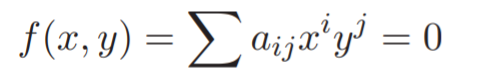# Algebraic Curve: Definition, Examples

An algebraic curve is any curve that can be defined by a polynomial equation .

More precisely, an algebraic curve has the polynomial equation:in x and y.
The degree of the algebraic curve is the degree of the polynomial f(x, y) .

The study of algebraic curves is one of the oldest fields of mathematics. The curves were widely used by the ancient Greeks, who used them to solve famous problems like the doubling of the cube (which requires solving the equation x3 = 2) or trisection of angles (which involves finding the intersection of a circle and a hyperbola) .

## Examples of Algebraic Curves

The familiar quadratic curves (e.g. circles, ellipses, and parabolas) are algebraic curves, as are a variety of cubic curves, quartic curves, and many other curves of higher degree.

The simplest example of an algebraic curve is the solution set of a two-variable polynomial equation in two dimensions; for example, a parabola defined by y = a(x – h)2. A shape like a spiral is not algebraic, as it cannot be defined by a polynomial. Trigonometric functions like the sine function are also not algebraic, as they also cannot be defined by a polynomial (thanks to that tricky “π”, which isn’t the root of any polynomial with integer coefficients).

When a curve is not algebraic, the curve (and the function that defines it) is transcendental. Some of these curves are defined by special functions.

## Defining the Algebraic Curve

The overview above describes the basic qualities of algebraic curves, but there are some unique characteristics that require a more precise definition. As the field has evolved over time, so has the multiplicity of language and symbols.

Algebraic curves are usually defined by complex numbers to ensure that the equations are unique. In math speak, that equates to a surface, or “objects of complex dimension 1.” Complex algebraic curves are orientable, which means they can be rotated in 3D space to produce spheres, donuts, and other surfaces. And they are usually (but not always) defined on the complex (affine) line . However, a large part of the theory of algebraic curves applies to rational numbers as well .

## References

 Degree of a Curve.
 Yan-Bin, J. (2017). Algebraic Curves. Retrieved Jan 22, 2022 from: https://faculty.sites.iastate.edu/jia/files/inline-files/algebraic-curves.pdf
 Algebraic Curves: A 2003 Math Circle Session by Renzo. Retrieved January 13, 2022 from: https://www.math.utah.edu/mathcircle/notes/curves.pdf
 Kerr, M. Algebraic Geometry III/IV.
 Gathmann, A. (2018). Plane Algebraic Curves: Class Notes TU Kaiserslautern. Retrieved Jan 22, 2022 from: https://www.mathematik.uni-kl.de/~gathmann/class/curves-2018/curves-2018.pdf

CITE THIS AS:
Stephanie Glen. "Algebraic Curve: Definition, Examples" From StatisticsHowTo.com: Elementary Statistics for the rest of us! https://www.statisticshowto.com/algebraic-curve/